### Home > GC > Chapter 11 > Lesson 11.2.1 > Problem11-77

11-77.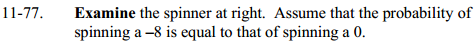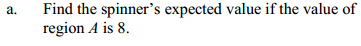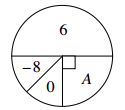Find the expected value of a single spin by finding the product of the probability and the value for each region. Find the sum for all the regions.

P(6) · (6) + P(−8) · (−8) + P(0) · (0) + P(A) · (8) =
expected value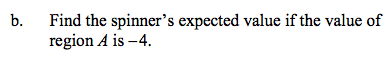Use the same equation from part (a), but put a −4 in place of the 8 for the value of A.

1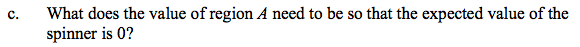Use the same equation from part (a), but put a 0 in place of the 8 for the value of A.

−8